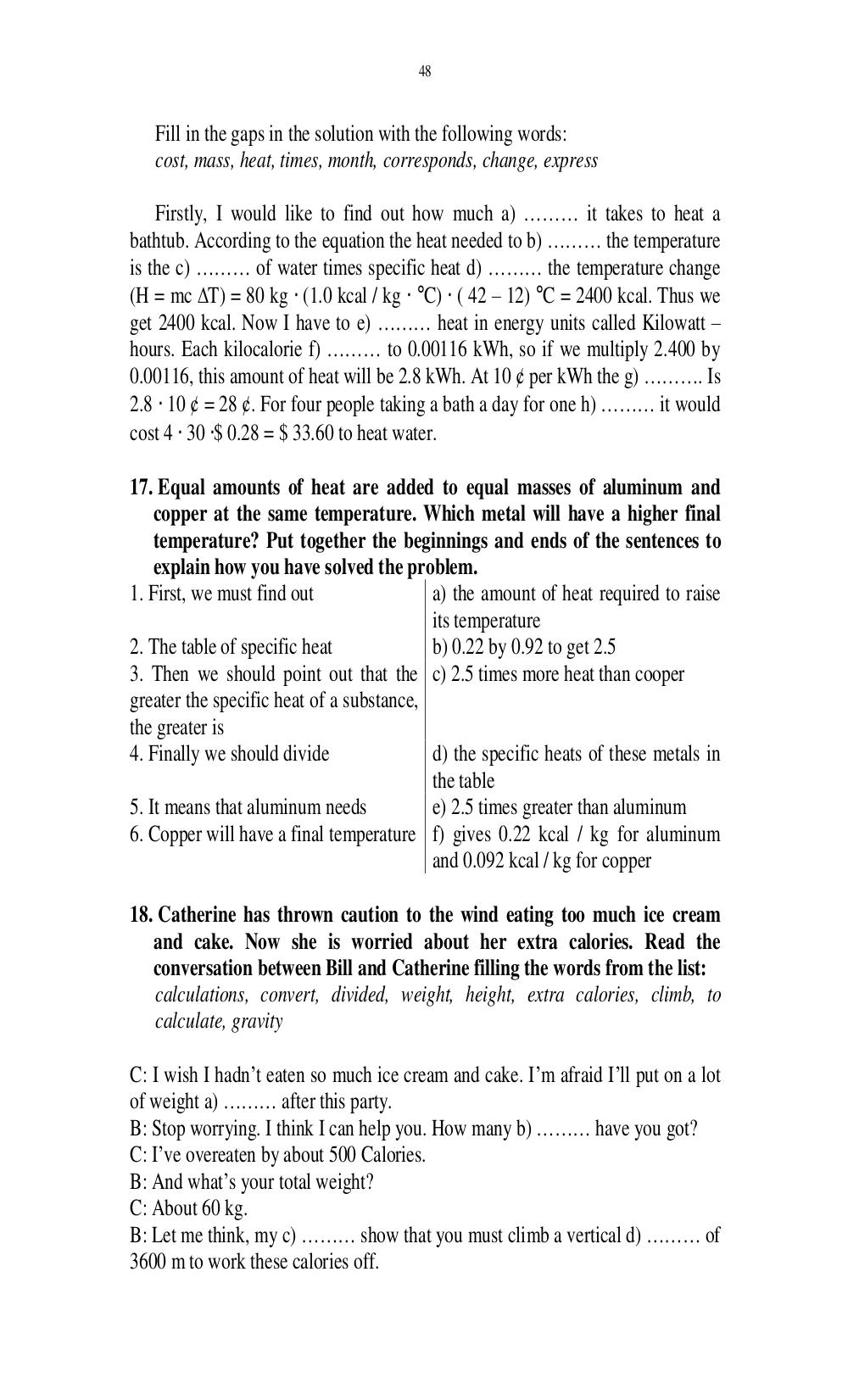# Английский язык. Ильичева Н.А - 48 стр.

Составители:

Рубрика:

• ## Иностранный язык48
Fill in the gaps in the solution with the following words:
cost, mass, heat, times, month, corresponds, change, express
Firstly, I would like to find out how much a) ……… it takes to heat a
bathtub. According to the equation the heat needed to b) …… the temperature
is the c) ……… of water times specific heat d) ……… the temperature change
(H = mc T) = 80 kg (1.0 kcal / kg °C) ( 42 12) °C = 2400 kcal. Thus we
get 2400 kcal. Now I have to e) …… heat in energy units called Kilowatt
hours. Each kilocalorie f) …… to 0.00116 kWh, so if we multiply 2.400 by
0.00116, this amount of heat will be 2.8 kWh. At 10 ¢ per kWh the g) ……. Is
2.8 10 ¢ = 28 ¢. For four people taking a bath a day for one h) ……… it would
cost 4 30 $0.28 =$ 33.60 to heat water.
17. Equal amounts of heat are added to equal masses of aluminum and
copper at the same temperature. Which metal will have a higher final
temperature? Put together the beginnings and ends of the sentences to
explain how you have solved the problem.
1. First, we must find out a) the amount of heat required to raise
its temperature
2. The table of specific heat b) 0.22 by 0.92 to get 2.5
3. Then we should point out that the
greater the specific heat of a substance,
the greater is
c) 2.5 times more heat than cooper
4. Finally we should divide d) the specific heats of these metals in
the table
5. It means that aluminum needs e) 2.5 times greater than aluminum
6. Copper will have a final temperature
f) gives 0.22 kcal / kg for aluminum
and 0.092 kcal / kg for copper
18. Catherine has thrown caution to the wind eating too much ice cream
and cake. Now she is worried about her extra calories. Read the
conversation between Bill and Catherine filling the words from the list:
calculations, convert, divided, weight, height, extra calories, climb, to
calculate, gravity
C: I wish I hadnt eaten so much ice cream and cake. Im afraid Ill put on a lot
of weight a) …… after this party.
B: Stop worrying. I think I can help you. How many b) ……… have you got?
C: Ive overeaten by about 500 Calories.
B: And whats your total weight?
B: Let me think, my c) ……… show that you must climb a vertical d) …… of
3600 m to work these calories off.48

Fill in the gaps in the solution with the following words:
cost, mass, heat, times, month, corresponds, change, express

Firstly, I would like to find out how much a) ……… it takes to heat a
bathtub. According to the equation the heat needed to b) ……… the temperature
is the c) ……… of water times specific heat d) ……… the temperature change
(H = mc ∆T) = 80 kg ⋅ (1.0 kcal / kg ⋅ °C) ⋅ ( 42 – 12) °C = 2400 kcal. Thus we
get 2400 kcal. Now I have to e) ……… heat in energy units called Kilowatt –
hours. Each kilocalorie f) ……… to 0.00116 kWh, so if we multiply 2.400 by
0.00116, this amount of heat will be 2.8 kWh. At 10 ¢ per kWh the g) ………. Is
2.8 ⋅ 10 ¢ = 28 ¢. For four people taking a bath a day for one h) ……… it would
cost 4 ⋅ 30 ⋅$0.28 =$ 33.60 to heat water.

17. Equal amounts of heat are added to equal masses of aluminum and
copper at the same temperature. Which metal will have a higher final
temperature? Put together the beginnings and ends of the sentences to
explain how you have solved the problem.
1. First, we must find out                a) the amount of heat required to raise
its temperature
2. The table of specific heat             b) 0.22 by 0.92 to get 2.5
3. Then we should point out that the c) 2.5 times more heat than cooper
greater the specific heat of a substance,
the greater is
4. Finally we should divide               d) the specific heats of these metals in
the table
5. It means that aluminum needs           e) 2.5 times greater than aluminum
6. Copper will have a final temperature f) gives 0.22 kcal / kg for aluminum
and 0.092 kcal / kg for copper

18. Catherine has thrown caution to the wind eating too much ice cream
and cake. Now she is worried about her extra calories. Read the
conversation between Bill and Catherine filling the words from the list:
calculations, convert, divided, weight, height, extra calories, climb, to
calculate, gravity

C: I wish I hadn’t eaten so much ice cream and cake. I’m afraid I’ll put on a lot
of weight a) ……… after this party.
B: Stop worrying. I think I can help you. How many b) ……… have you got?
C: I’ve overeaten by about 500 Calories.
B: And what’s your total weight?